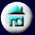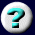17. The Drive To Make Things       Happen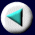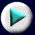Introduction
 When we think about the force of gravity, and the spontaneous tendency for a stream to flow or a ball to roll downhill, it is convenient to regard it as a tendency for the water or the ball to go from a region of high to low potential energy. Only when such a drop in potential is possible can useful work be obtained from the process. The attractive force that the Earth exerts on a ball of mass m is F = mg, in which g is the gravitational constant. If we lift the ball to a height h above some starting level, we give it an extra potential energy of Ep = mgh. The ball can convert this potential energy to kinetic energy of motion by rolling downhill to the original level, as represented on the next page. Spontaneous motion occurs from a region of high potential to one of low potential. The same language is useful in studying chemical reactions. We know that a spontaneous chemical reaction at constant overall temperature and pressure is one that leads to a decrease in free energy, G. The combination of hydrogen with oxygen to form water is highly spontaneous, and can be explosively fast: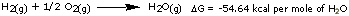Page 1 of 56